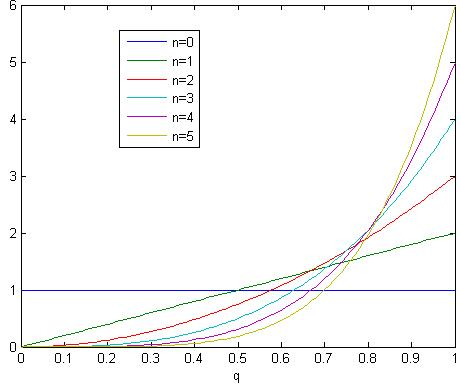### Archive

Archive for the ‘math’ Category

## Recommend 3blue1brown

April 24th, 2018

When I was in high school and saw the following equation, my mind was blown!Why is Pi here? Isn’t it supposed to show up only in circle related problem? But the left-hand has nothing to do with circle. And it’s Pi squared!

Even today I still do not have an intuitive understanding why the above equation is true, until I watched a visual explanation from 3blue1brown. This video provide an elegant and visual proof of the equation. Go ahead and watch it:

Xu Cui Categories: Tags:

## What is the probability that the sun will rise tomorrow?

May 6th, 2011

Laplace introduced the sunrise problem in 18th century. With prior knowledge that the sun has risen N days in the past consecutively (not knowing the gravity rules etc), what is your confidence that the sun will rise tomorrow?

Let p be the probability that the sun rise. Apparently p range from 0 to 1. Without any prior knowledge, we have same level of confidence to believe p=0, 0.1, 0.2, …, or 1. So we assume equal probability distribution on p.

Now assume day 1 passed and we found the sun rose. Based on this knowledge, how should we update our belief on p? Well, p=0 is impossible, otherwise we will have not observed the sunrise. p=0.1 is possible but unlikely. Because if p=0.1 we only have 10% probability to observe a sunrise but we did observe one. We can actually formally calculate P(p=q|N=1) = P(N=1|p=q) * P(p=q) / P(N=1) using Bayes’s theorem. Since p(N=1) is in the denominator and it doesn’t depend on q, we don’t care. Our prior belief of P(p=q) was a uniform distribution. P(N=1|p=q) is equal to q. So the result is P(p=q|N=1) = q. After normalization (i.e. our total ‘belief’ over all p should be 1), P(p=q|N=1) = 2*q. So we have updated our belief from uniform distribution to a skewed distribution towards bigger probability of sunrise.

Based on our new belief (i.e. P(p=q)=2*q), what is our confidence that the sun will rise tomorrow? We simply need to sum (or integrate) all the possibilities. The final result equals to int(2*q^2) = 2/3. Yes, we have 2/3 confidence to say the sun will rise tomorrow, given we have observed one sunrise.

We then continue doing this for N=2, 3, …. The confidence level equals to (N+1)/(N+2). If we have observed sunrise for 1000 days, our confidence level raised to 0.999.The belief of the probability of sunrise based on n observations of sunrise

But if we have prior knowledge of the gravity rules, our belief will be dramatically different.

Xu Cui Categories: math Tags: